# AlgebraPage 2

#### WATCH ALL SLIDES

Slide 17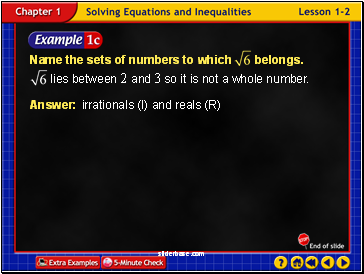Example 2-1c

Answer: irrationals (I) and reals (R)

Slide 18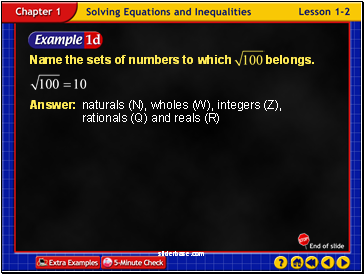Example 2-1d

Answer: naturals (N), wholes (W), integers (Z), rationals (Q) and reals (R)

Slide 19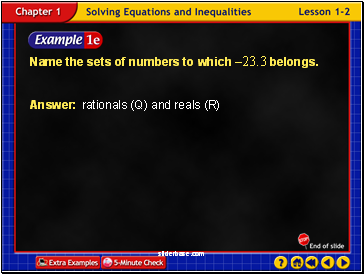Example 2-1e

Answer: rationals (Q) and reals (R)

Name the sets of numbers to which –23.3 belongs.

Slide 20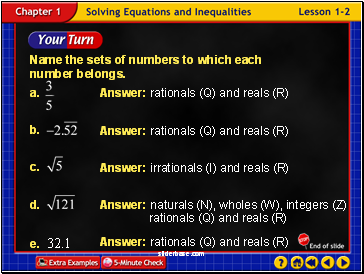Example 2-1f

Answer: rationals (Q) and reals (R)

Answer: rationals (Q) and reals (R)

Answer: irrationals (I) and reals (R)

Answer: naturals (N), wholes (W), integers (Z) rationals (Q) and reals (R)

Answer: rationals (Q) and reals (R)

Slide 21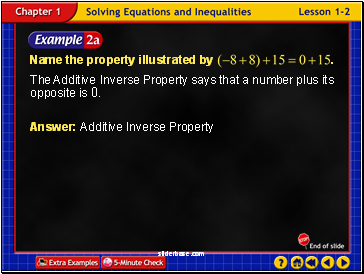Example 2-2a

The Additive Inverse Property says that a number plus its opposite is 0.

Slide 22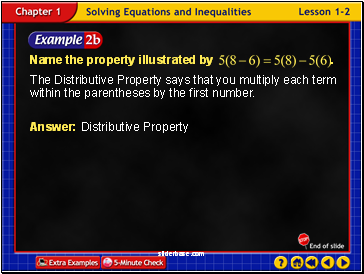Example 2-2b

The Distributive Property says that you multiply each term within the parentheses by the first number.

Slide 23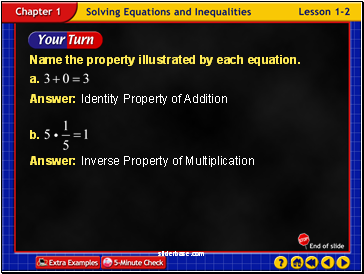Example 2-2c

Slide 24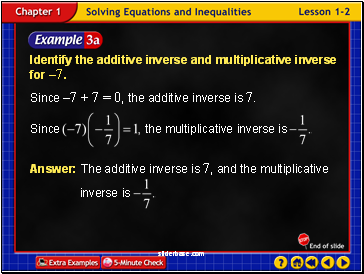Example 2-3a

Identify the additive inverse and multiplicative inverse for –7.

Since –7 + 7 = 0, the additive inverse is 7.

Slide 25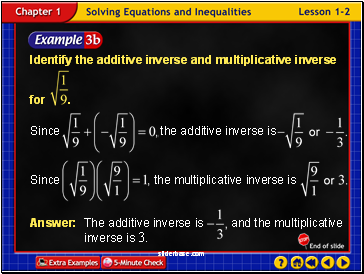Example 2-3b

Slide 26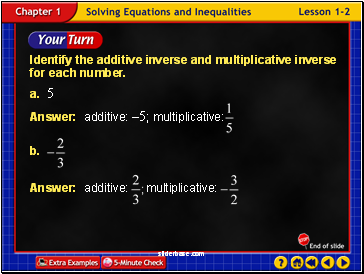Example 2-3c

Slide 27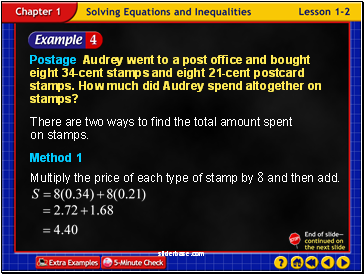Example 2-4a

Postage Audrey went to a post office and bought eight 34-cent stamps and eight 21-cent postcard stamps. How much did Audrey spend altogether on stamps?

There are two ways to find the total amount spent on stamps.

Method 1

Multiply the price of each type of stamp by 8 and then add.

Slide 28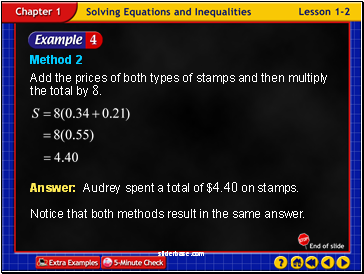Example 2-4b

Method 2

Add the prices of both types of stamps and then multiply the total by 8.

Answer: Audrey spent a total of \$4.40 on stamps.

Notice that both methods result in the same answer.

Slide 29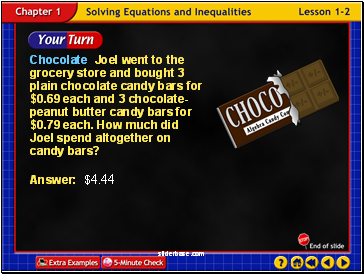Example 2-4c

Chocolate Joel went to the grocery store and bought 3 plain chocolate candy bars for \$0.69 each and 3 chocolate-peanut butter candy bars for \$0.79 each. How much did Joel spend altogether on candy bars?

Go to page:
1  2  3  4  5  6  7  8